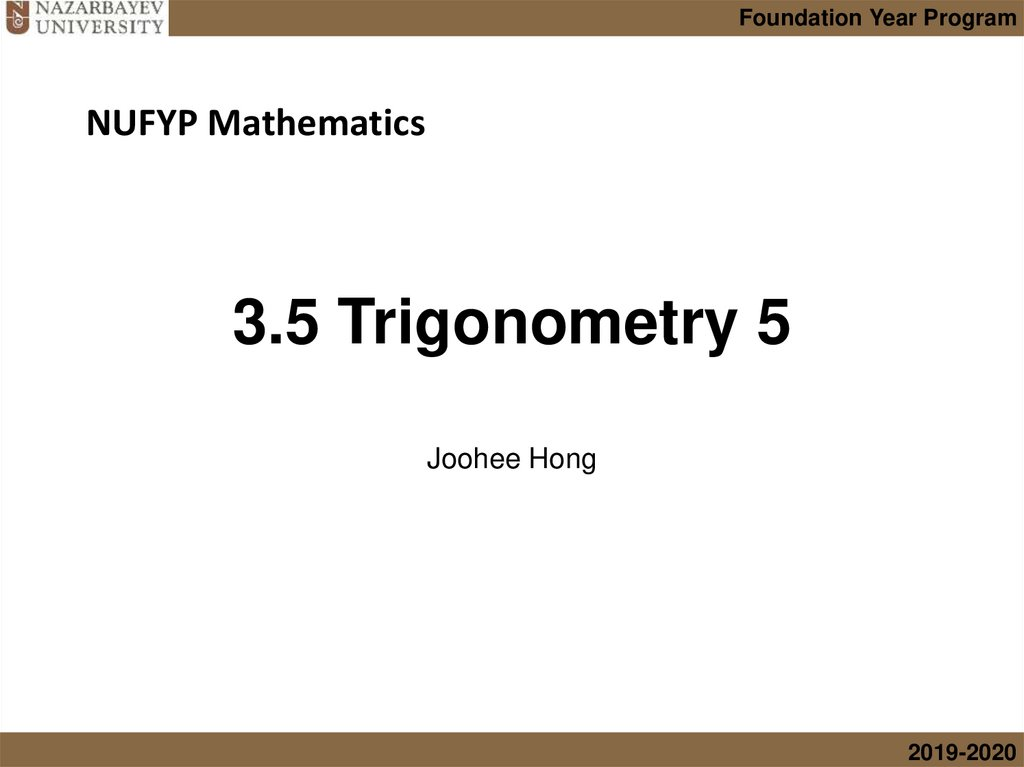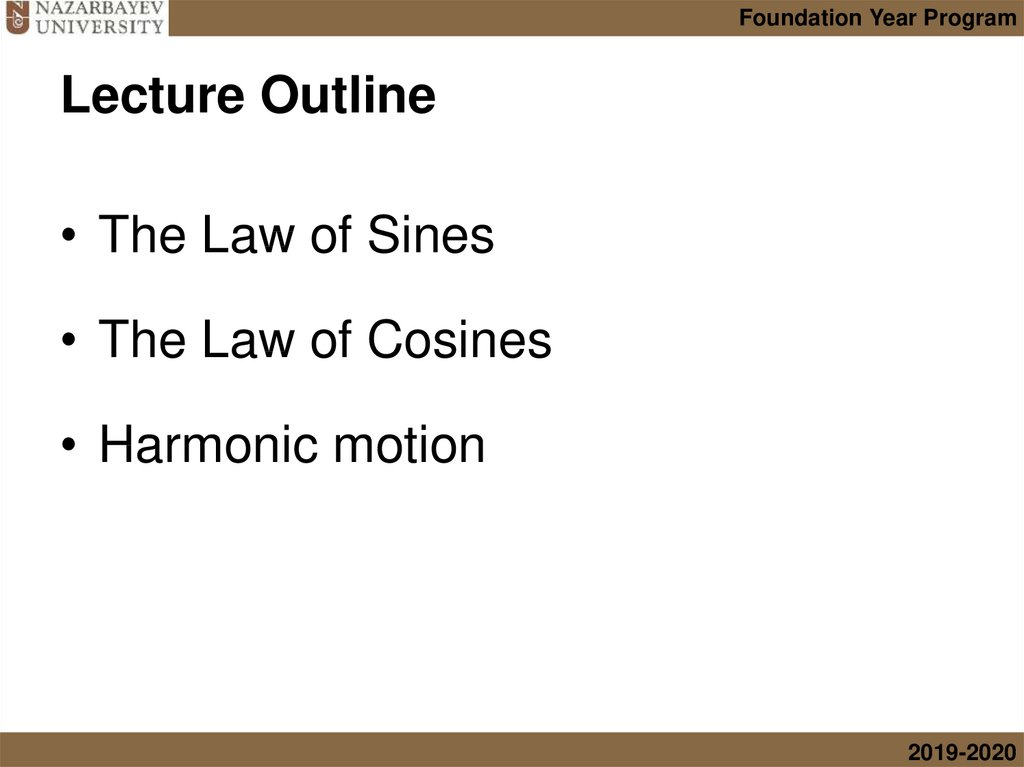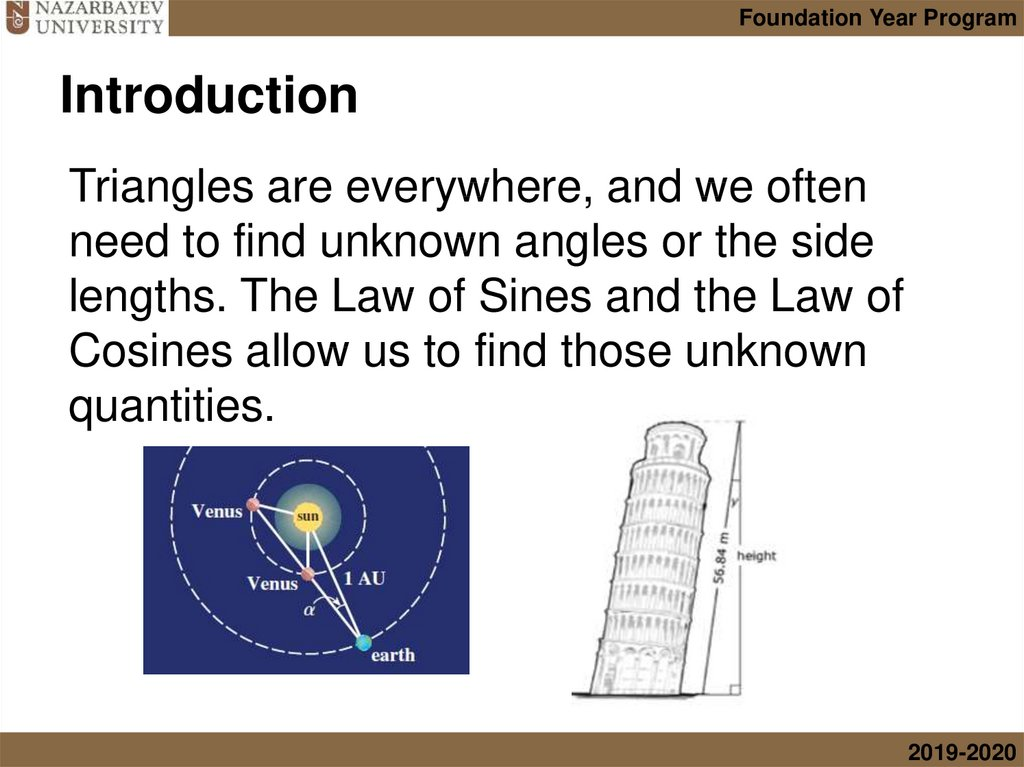# 3.5 Trigonometry 5

## 1. 3.5 Trigonometry 5

Foundation Year Program
NUFYP Mathematics
3.5 Trigonometry 5
Joohee Hong
2019-2020

## 2. Lecture Outline

Foundation Year Program
Lecture Outline
• The Law of Sines
• The Law of Cosines
• Harmonic motion
2019-2020

## 3. Introduction

Foundation Year Program
Introduction
Triangles are everywhere, and we often
need to find unknown angles or the side
lengths. The Law of Sines and the Law of
Cosines allow us to find those unknown
quantities.
2019-2020

## 4. The Law of Sines

Foundation Year Program
The Law of Sines
• The Law of Sines says that in any triangle
the lengths of the sides are proportional to
the sines of the corresponding opposite
angles. That is,
sin